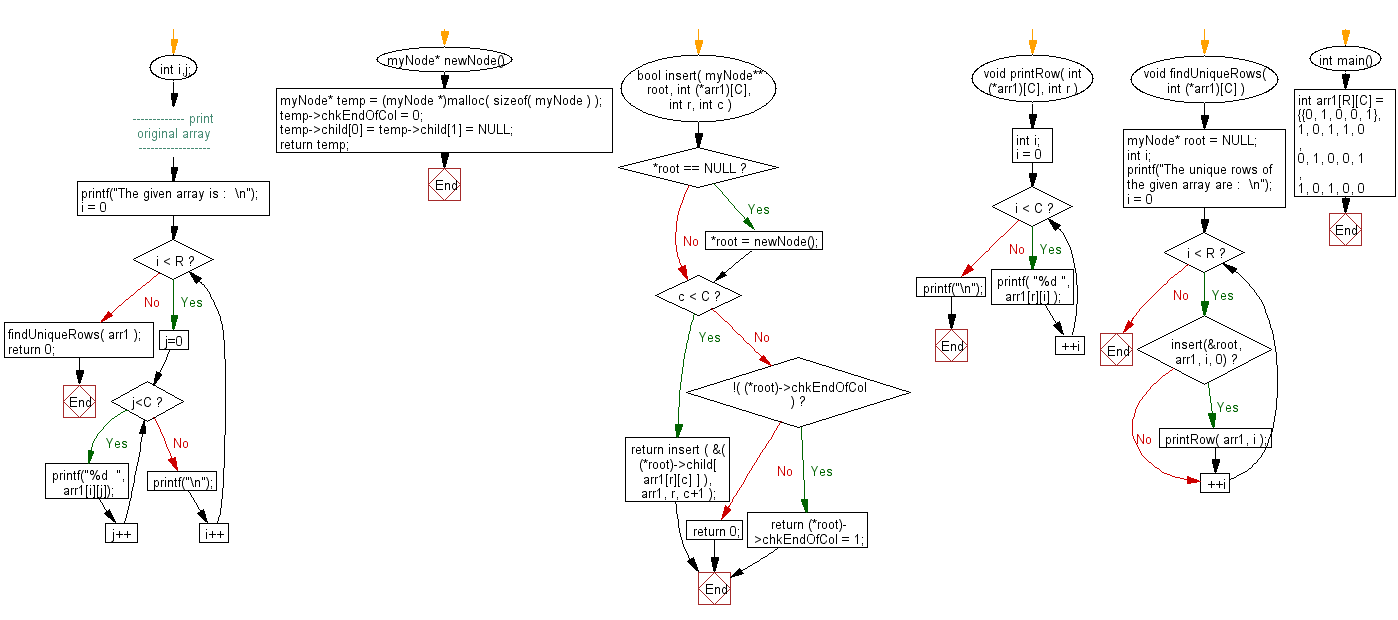﻿ C exercises: Return only the unique rows from a given binary matrix - w3resource# C Exercises: Return only the unique rows from a given binary matrix

## C Array: Exercise-72 with Solution

Write a program in C to return only the unique rows from a given binary matrix.

Sample Solution:

C Code:

``````#include <stdio.h>
#include <stdlib.h>
#include <stdbool.h>
#define R 4
#define C 5

typedef struct Node
{
bool chkEndOfCol;
struct Node *child;
} myNode;
myNode* newNode()
{
myNode* temp = (myNode *)malloc( sizeof( myNode ) );
temp->chkEndOfCol = 0;
temp->child = temp->child = NULL;
return temp;
}
bool insert( myNode** root, int (*arr1)[C], int r, int c )
{
if ( *root == NULL )
*root = newNode();
if ( c < C )
return insert ( &( (*root)->child[ arr1[r][c] ] ), arr1, r, c+1 );
else
{
if ( !( (*root)->chkEndOfCol ) )
return (*root)->chkEndOfCol = 1;
return 0;
}
}
void printRow( int (*arr1)[C], int r )
{
int i;
for( i = 0; i < C; ++i )
printf( "%d ", arr1[r][i] );
printf("\n");
}
void findUniqueRows( int (*arr1)[C] )
{
myNode* root = NULL;
int i;
printf("The unique rows of the given array are :  \n");
for ( i = 0; i < R; ++i )
if ( insert(&root, arr1, i, 0) )
printRow( arr1, i );
}
int main()
{
int arr1[R][C] =
{{0, 1, 0, 0, 1},
{1, 0, 1, 1, 0},
{0, 1, 0, 0, 1},
{1, 0, 1, 0, 0}
};
int i,j;
//------------- print original array ------------------
printf("The given array is :  \n");
for(i = 0; i < R; i++)
{
for (j=0;j<C;j++)
{
printf("%d  ", arr1[i][j]);
}
printf("\n");
}
//------------------------------------------------------

findUniqueRows( arr1 );
return 0;
}
```
```

Sample Output:

```The given array is :
0  1  0  0  1
1  0  1  1  0
0  1  0  0  1
1  0  1  0  0
The unique rows of the given array are :
0 1 0 0 1
1 0 1 1 0
1 0 1 0 0
```

Flowchart:C Programming Code Editor:

Improve this sample solution and post your code through Disqus.

What is the difficulty level of this exercise?

﻿